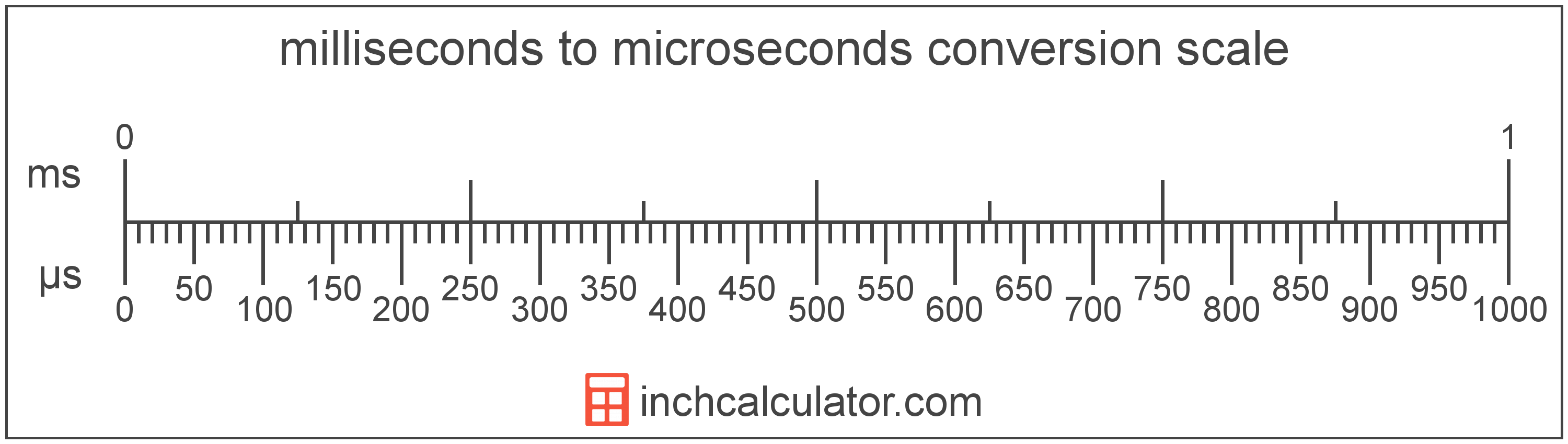# Milliseconds to Microseconds Converter

Enter the time in milliseconds below to get the value converted to microseconds.

Results in Microseconds:1 ms = 1,000 µs

Do you want to convert microseconds to milliseconds?

## How to Convert Milliseconds to Microseconds

To convert a measurement in milliseconds to a measurement in microseconds, multiply the time by the following conversion ratio: 1,000 microseconds/millisecond.

Since one millisecond is equal to 1,000 microseconds, you can use this simple formula to convert:

microseconds = milliseconds × 1,000

The time in microseconds is equal to the time in milliseconds multiplied by 1,000.

For example, here's how to convert 5 milliseconds to microseconds using the formula above.
microseconds = (5 ms × 1,000) = 5,000 µs### How Many Microseconds Are in a Millisecond?

There are 1,000 microseconds in a millisecond, which is why we use this value in the formula above.

1 ms = 1,000 µs

## What Is a Millisecond?

One millisecond is equal to 1/1,000 of a second or 1,000 microseconds.

The millisecond is a multiple of the second, which is the SI base unit for time. In the metric system, "milli" is the prefix for thousandths, or 10-3. Milliseconds can be abbreviated as ms; for example, 1 millisecond can be written as 1 ms.

## What Is a Microsecond?

One microsecond is equal to 1/1,000 of a millisecond or 1,000 nanoseconds.

The microsecond is a multiple of the second, which is the SI base unit for time. In the metric system, "micro" is the prefix for millionths, or 10-6. Microseconds can be abbreviated as µs; for example, 1 microsecond can be written as 1 µs.

## Millisecond to Microsecond Conversion Table

Table showing various millisecond measurements converted to microseconds.
Milliseconds Microseconds
0.001 ms 1 µs
0.002 ms 2 µs
0.003 ms 3 µs
0.004 ms 4 µs
0.005 ms 5 µs
0.006 ms 6 µs
0.007 ms 7 µs
0.008 ms 8 µs
0.009 ms 9 µs
0.01 ms 10 µs
0.02 ms 20 µs
0.03 ms 30 µs
0.04 ms 40 µs
0.05 ms 50 µs
0.06 ms 60 µs
0.07 ms 70 µs
0.08 ms 80 µs
0.09 ms 90 µs
0.1 ms 100 µs
0.2 ms 200 µs
0.3 ms 300 µs
0.4 ms 400 µs
0.5 ms 500 µs
0.6 ms 600 µs
0.7 ms 700 µs
0.8 ms 800 µs
0.9 ms 900 µs
1 ms 1,000 µs